Home    |    Teacher    |    Parents    |    Glossary    |    About UsThere are many different kinds of quadrilaterals, but all have several things in common: all of them have four sides, are coplanar, have two diagonals, and the sum of their four interior angles equals 360 degrees. This is how they are alike, but what makes them different?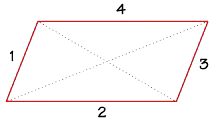We know many quadrilaterals by their special shapes and properties, like squares. Remember, if you see the word quadrilateral, it does not necessarily mean a figure with special properties like a square or rectangle! In word problems, be careful not to assume that a quadrilateral has parallel sides or equal sides unless that is stated.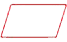A parallelogram has two parallel pairs of opposite sides.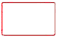A rectangle has two pairs of opposite sides parallel, and four right angles. It is also a parallelogram, since it has two pairs of parallel sides.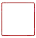A square has two pairs of parallel sides, four right angles, and all four sides are equal. It is also a rectangle and a parallelogram.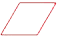A rhombus is defined as a parallelogram with four equal sides. Is a rhombus always a rectangle? No, because a rhombus does not have to have 4 right angles.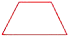Trapezoids only have one pair of parallel sides. It's a type of quadrilateral that is not a parallelogram. (British name: Trapezium)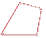Kites have two pairs of adjacent sides that are equal.

We can use a Venn diagram to help us group the types of quadrilaterals.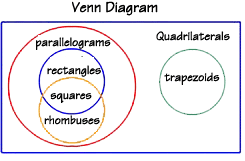A Venn diagram uses overlapping circles to show relationships between groups of objects. All "quadrilaterals" can be separated into three sub-groups: general quadrilaterals, parallelograms and trapezoids.

Is a rectangle always a rhombus? No, because all four sides of a rectangle don't have to be equal. However, the sets of rectangles and rhombuses do intersect, and their intersection is the set of squares—all squares are both a rectangle and a rhombus.

We can put squares in the intersection of the two circles.

From this diagram, you can see that a square is a quadrilateral, a parallelogram, a rectangle, and a rhombus!

Is a trapezoid a parallelogram? No, because a trapezoid has only one pair of parallel sides. That is why we must show the set of trapezoids in a separate circle on the Venn diagram.

What about kites? Kites are quadrilaterals that can be parallelograms. If their two pairs of sides are equal, it becomes a rhombus, and if their angles are equal, it becomes a square.

 Homework Help | Geometry | PolygonsEmail this page to a friendSearch·  Polygon basics·  Triangles·  Quadrilaterals·  Area of polygons     and circlesFirst Glance In Depth Examples WorkoutQuadrilaterals